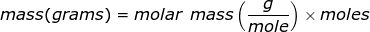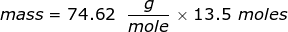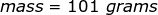# Molar Mass Formula

Molar Mass Formula
Molar mass is the mass of one mole of a substance. The mass will be represented in grams while a mole of a substance is defined as 6.02 x 1023 representative particles (Avogadro's number) of that substance.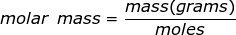Molar Mass Formula Questions:

1. Calculate the molar mass of a substance if 0.235 moles has a mass of 45.67 grams.2. Calculate the number of grams of a substance if 1.35 moles has a molar mass of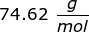.

The equation of molar mass will need to be rearranged to solve for the mass.